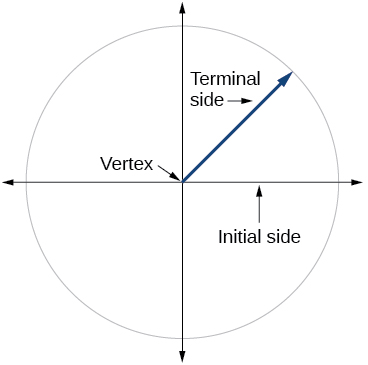# 7.1 Angles  (Page 9/29)

 Page 9 / 29

A satellite is rotating around Earth at 0.25 radian per hour at an altitude of 242 km above Earth. If the radius of Earth is 6378 kilometers, find the linear speed of the satellite in kilometers per hour.

1655 kilometers per hour

## Key equations

 arc length $s=r\theta$ area of a sector $A=\frac{1}{2}\theta {r}^{2}$ angular speed $\omega =\frac{\theta }{t}$ linear speed $v=\frac{s}{t}$ linear speed related to angular speed $v=r\omega$

## Key concepts

• An angle is formed from the union of two rays, by keeping the initial side fixed and rotating the terminal side. The amount of rotation determines the measure of the angle.
• An angle is in standard position if its vertex is at the origin and its initial side lies along the positive x -axis. A positive angle is measured counterclockwise from the initial side and a negative angle is measured clockwise.
• To draw an angle in standard position, draw the initial side along the positive x -axis and then place the terminal side according to the fraction of a full rotation the angle represents. See [link] .
• In addition to degrees, the measure of an angle can be described in radians. See [link] .
• To convert between degrees and radians, use the proportion $\text{\hspace{0.17em}}\frac{\theta }{180}=\frac{{\theta }^{R}}{\pi }.\text{\hspace{0.17em}}$ See [link] and [link] .
• Two angles that have the same terminal side are called coterminal angles.
• We can find coterminal angles by adding or subtracting $\text{\hspace{0.17em}}360°\text{\hspace{0.17em}}$ or $\text{\hspace{0.17em}}2\pi .\text{\hspace{0.17em}}$ See [link] and [link] .
• Coterminal angles can be found using radians just as they are for degrees. See [link] .
• The length of a circular arc is a fraction of the circumference of the entire circle. See [link] .
• The area of sector is a fraction of the area of the entire circle. See [link] .
• An object moving in a circular path has both linear and angular speed.
• The angular speed of an object traveling in a circular path is the measure of the angle through which it turns in a unit of time. See [link] .
• The linear speed of an object traveling along a circular path is the distance it travels in a unit of time. See [link] .

## Verbal

Draw an angle in standard position. Label the vertex, initial side, and terminal side.Explain why there are an infinite number of angles that are coterminal to a certain angle.

State what a positive or negative angle signifies, and explain how to draw each.

Whether the angle is positive or negative determines the direction. A positive angle is drawn in the counterclockwise direction, and a negative angle is drawn in the clockwise direction.

How does radian measure of an angle compare to the degree measure? Include an explanation of 1 radian in your paragraph.

Explain the differences between linear speed and angular speed when describing motion along a circular path.

Linear speed is a measurement found by calculating distance of an arc compared to time. Angular speed is a measurement found by calculating the angle of an arc compared to time.

#### Questions & Answers

write down the polynomial function with root 1/3,2,-3 with solution
if A and B are subspaces of V prove that (A+B)/B=A/(A-B)
write down the value of each of the following in surd form a)cos(-65°) b)sin(-180°)c)tan(225°)d)tan(135°)
Prove that (sinA/1-cosA - 1-cosA/sinA) (cosA/1-sinA - 1-sinA/cosA) = 4
what is the answer to dividing negative index
In a triangle ABC prove that. (b+c)cosA+(c+a)cosB+(a+b)cisC=a+b+c.
give me the waec 2019 questions
the polar co-ordinate of the point (-1, -1)
prove the identites sin x ( 1+ tan x )+ cos x ( 1+ cot x )= sec x + cosec x
tanh`(x-iy) =A+iB, find A and B
B=Ai-itan(hx-hiy)
Rukmini
what is the addition of 101011 with 101010
If those numbers are binary, it's 1010101. If they are base 10, it's 202021.
Jack
extra power 4 minus 5 x cube + 7 x square minus 5 x + 1 equal to zero
the gradient function of a curve is 2x+4 and the curve passes through point (1,4) find the equation of the curve
1+cos²A/cos²A=2cosec²A-1
test for convergence the series 1+x/2+2!/9x3ByByByBy